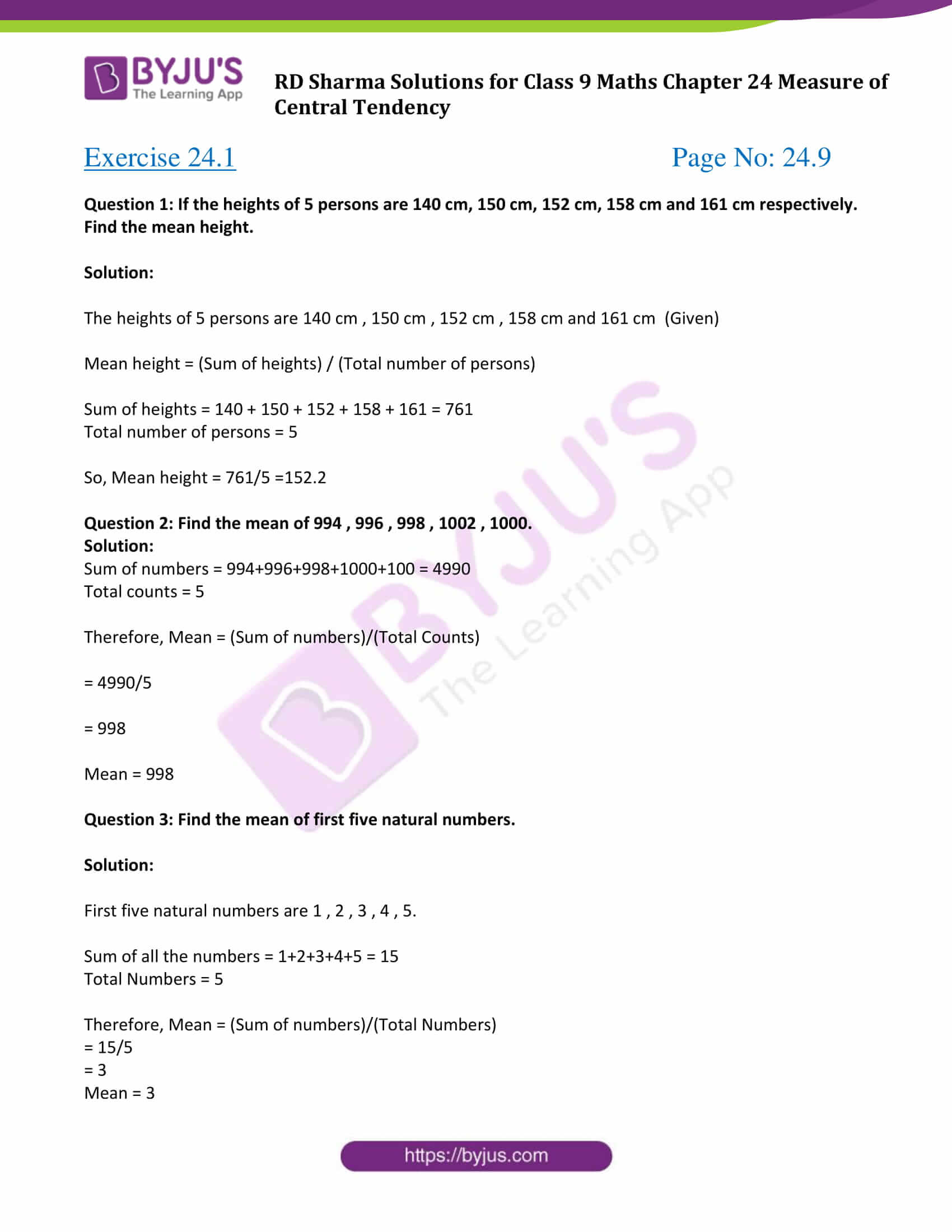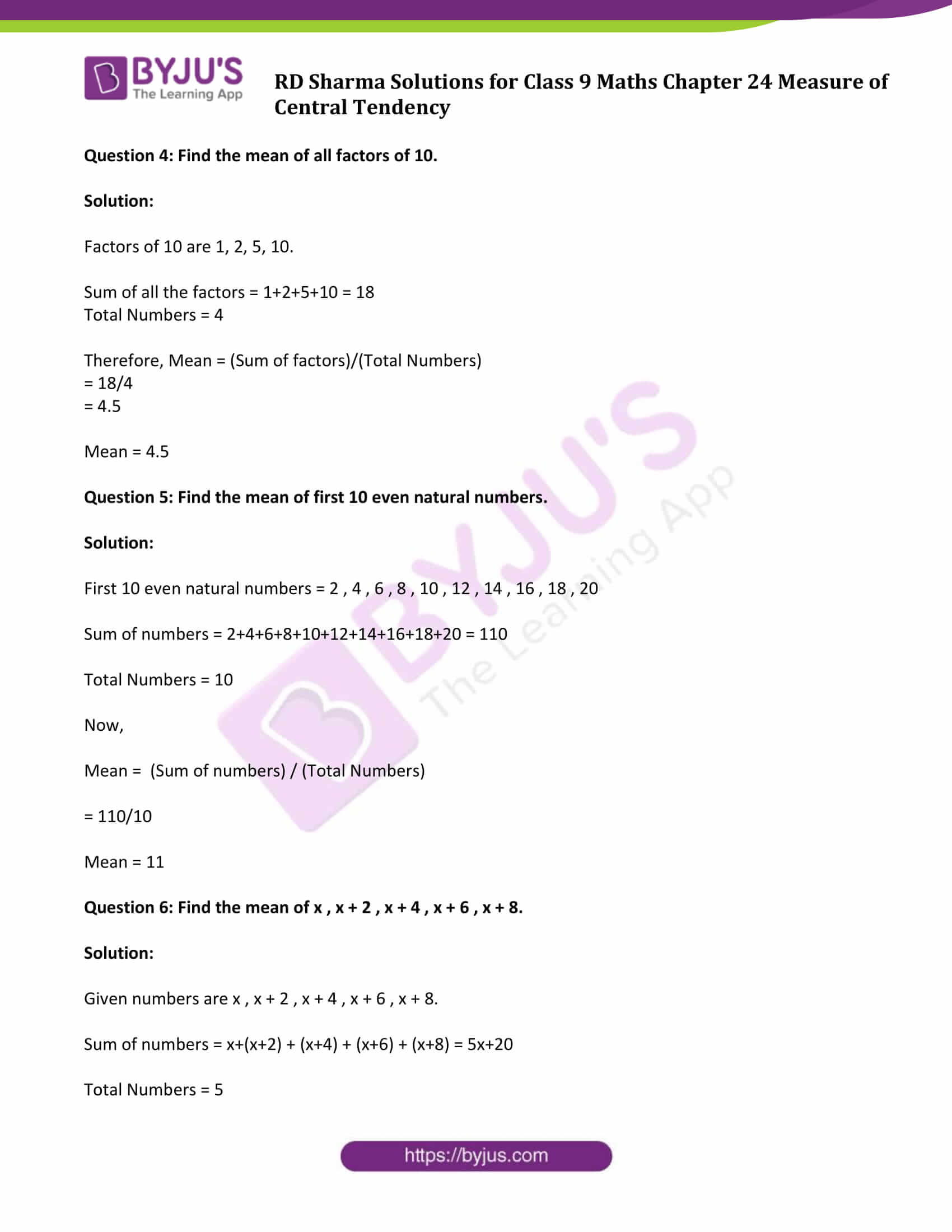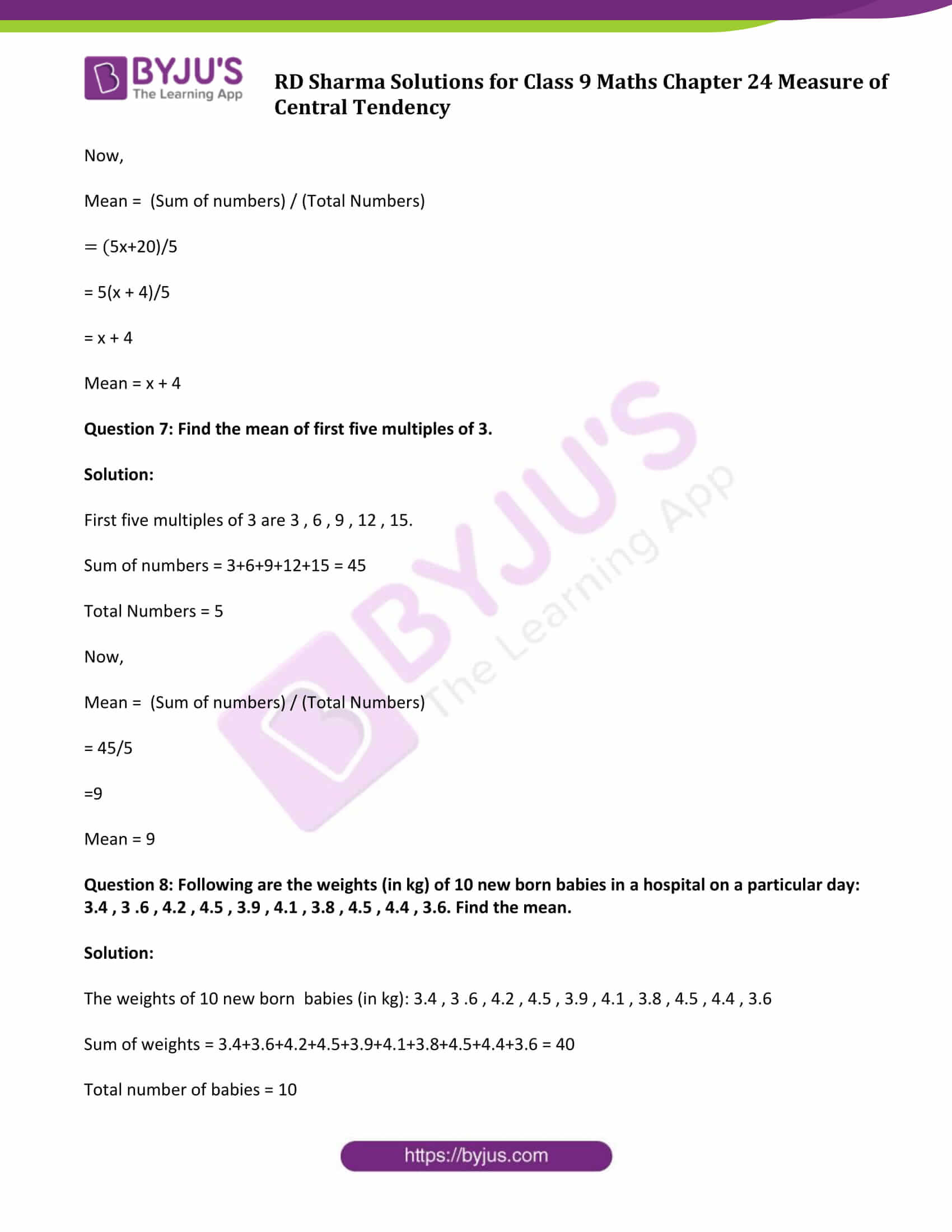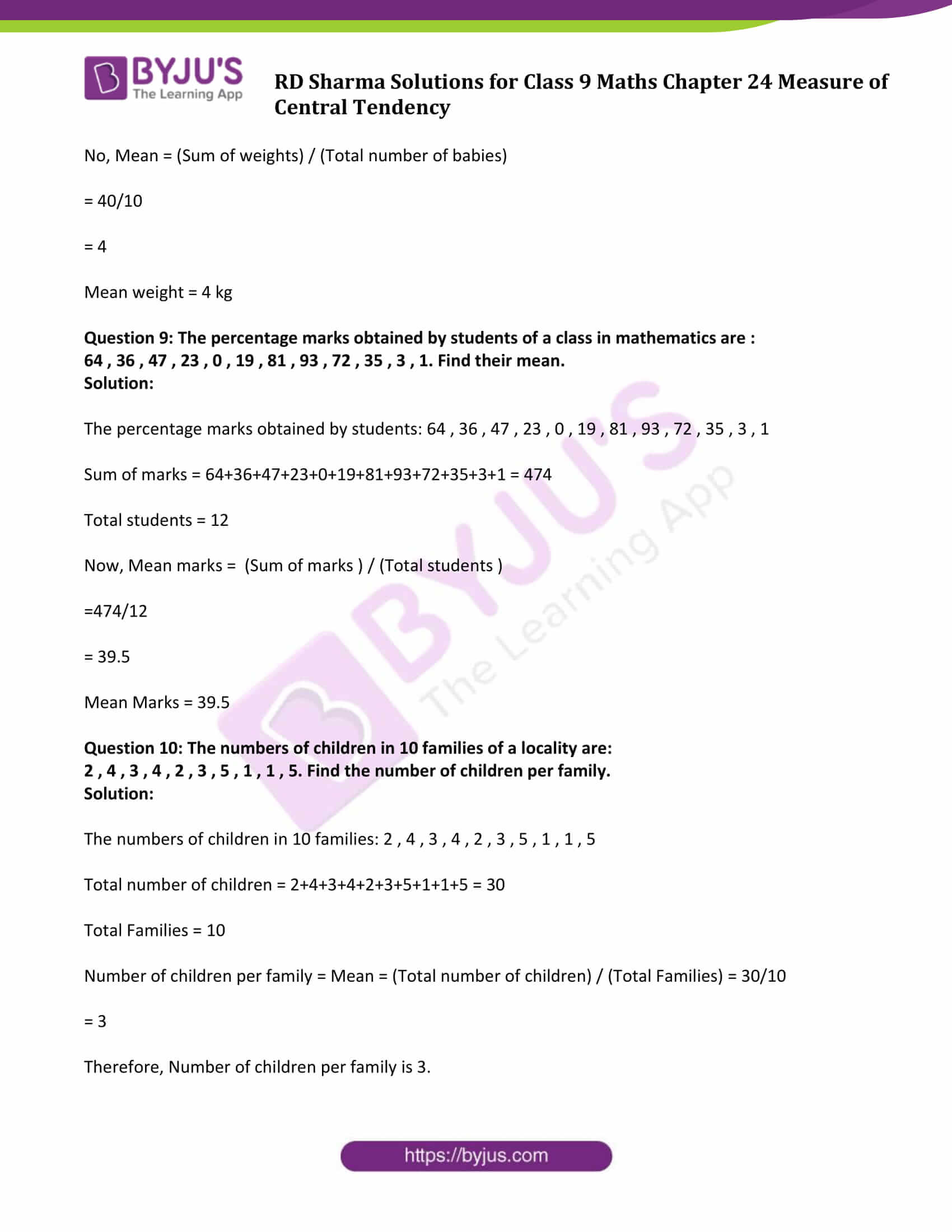# RD Sharma Solutions for Class 9 Maths Chapter 24 Measure of Central Tendency Exercise 24.1

Class 9 Chapter 24 – Measure of Central Tendency Exercise 24.1 solutions are provided here. RD Sharma Class 9 textbook offers a much harder approach to questions of Measures of Central Tendency when compared to the other textbooks. Solving the RD Sharma questions requires you to have an extensive understanding of the topic in hand. Each question is solved by subject experts in an easy language and given step-by-step solutions for better understanding. This exercise problems help students to solve the arithmetic mean of given data. Download the pdf now and start practicing.

## Download PDF of RD Sharma Solutions for Class 9 Maths Chapter 24 Measure of Central Tendency Exercise 24.1### Access Answers to Maths RD Sharma Solutions for Class 9 Chapter 24 Measure of Central Tendency Exercise 24.1 Page number 24.9

Question 1: If the heights of 5 persons are 140 cm, 150 cm, 152 cm, 158 cm and 161 cm respectively. Find the mean height.

Solution:

The heights of 5 persons are 140 cm , 150 cm , 152 cm , 158 cm and 161 cm (Given)

Mean height = (Sum of heights) / (Total number of persons)

Sum of heights = 140 + 150 + 152 + 158 + 161 = 761

Total number of persons = 5

So, Mean height = 761/5 =152.2

Question 2: Find the mean of 994 , 996 , 998 , 1002 , 1000.

Solution:

Sum of numbers = 994+996+998+1000+100 = 4990

Total counts = 5

Therefore, Mean = (Sum of numbers)/(Total Counts)

= 4990/5

= 998

Mean = 998

Question 3: Find the mean of first five natural numbers.

Solution:

First five natural numbers are 1 , 2 , 3 , 4 , 5.

Sum of all the numbers = 1+2+3+4+5 = 15

Total Numbers = 5

Therefore, Mean = (Sum of numbers)/(Total Numbers)

= 15/5

= 3

Mean = 3

Question 4: Find the mean of all factors of 10.

Solution:

Factors of 10 are 1, 2, 5, 10.

Sum of all the factors = 1+2+5+10 = 18

Total Numbers = 4

Therefore, Mean = (Sum of factors)/(Total Numbers)

= 18/4

= 4.5

Mean = 4.5

Question 5: Find the mean of first 10 even natural numbers.

Solution:

First 10 even natural numbers = 2 , 4 , 6 , 8 , 10 , 12 , 14 , 16 , 18 , 20

Sum of numbers = 2+4+6+8+10+12+14+16+18+20 = 110

Total Numbers = 10

Now,

Mean = (Sum of numbers) / (Total Numbers)

= 110/10

Mean = 11

Question 6: Find the mean of x , x + 2 , x + 4 , x + 6 , x + 8.

Solution:

Given numbers are x , x + 2 , x + 4 , x + 6 , x + 8.

Sum of numbers = x+(x+2) + (x+4) + (x+6) + (x+8) = 5x+20

Total Numbers = 5

Now,

Mean = (Sum of numbers) / (Total Numbers)

= (5x+20)/5

= 5(x + 4)/5

= x + 4

Mean = x + 4

Question 7: Find the mean of first five multiples of 3.

Solution:

First five multiples of 3 are 3 , 6 , 9 , 12 , 15.

Sum of numbers = 3+6+9+12+15 = 45

Total Numbers = 5

Now,

Mean = (Sum of numbers) / (Total Numbers)

= 45/5

=9

Mean = 9

Question 8: Following are the weights (in kg) of 10 new born babies in a hospital on a particular day: 3.4 , 3 .6 , 4.2 , 4.5 , 3.9 , 4.1 , 3.8 , 4.5 , 4.4 , 3.6. Find the mean.

Solution:

The weights of 10 new born babies (in kg): 3.4 , 3 .6 , 4.2 , 4.5 , 3.9 , 4.1 , 3.8 , 4.5 , 4.4 , 3.6

Sum of weights = 3.4+3.6+4.2+4.5+3.9+4.1+3.8+4.5+4.4+3.6 = 40

Total number of babies = 10

No, Mean = (Sum of weights) / (Total number of babies)

= 40/10

= 4

Mean weight = 4 kg

Question 9: The percentage marks obtained by students of a class in mathematics are :
64 , 36 , 47 , 23 , 0 , 19 , 81 , 93 , 72 , 35 , 3 , 1. Find their mean.

Solution:

The percentage marks obtained by students: 64 , 36 , 47 , 23 , 0 , 19 , 81 , 93 , 72 , 35 , 3 , 1

Sum of marks = 64+36+47+23+0+19+81+93+72+35+3+1 = 474

Total students = 12

Now, Mean marks = (Sum of marks ) / (Total students )

=474/12

= 39.5

Mean Marks = 39.5

Question 10: The numbers of children in 10 families of a locality are:

2 , 4 , 3 , 4 , 2 , 3 , 5 , 1 , 1 , 5. Find the number of children per family.

Solution:

The numbers of children in 10 families: 2 , 4 , 3 , 4 , 2 , 3 , 5 , 1 , 1 , 5

Total number of children = 2+4+3+4+2+3+5+1+1+5 = 30

Total Families = 10

Number of children per family = Mean = (Total number of children) / (Total Families) = 30/10

= 3

Therefore, Number of children per family is 3.

## RD Sharma Solutions for Class 9 Maths Chapter 24 Measures of Central Tendency Exercise 24.1

RD Sharma Solutions Class 9 Maths Chapter 24 Measures of Central Tendency Exercise 24.1 is based on the following topics:

• Measure of Central Tendency Introduction
• Arithmetic mean of individual observations or ungrouped data
• Properties of arithmetic mean